# Data Aggregation

Data Aggregation groups raw data points into points with a larger argument measurement unit. For example, if data points’ arguments are measured in minutes, data aggregation can create points measured in hours or days to display fewer points.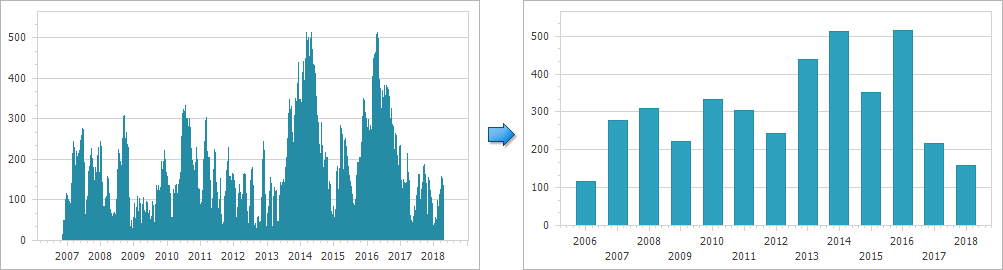When the data aggregation is enabled, the X-axis splits its range into intervals and automatically aggregates data for each interval using the aggregate function. For example, the title image shows chart data aggregated by Years using the Average aggregate function.

Important

Data aggregation is available for the argument axis (X-axis) only.

Data aggregation is available depending on the X-Axis’s scale type and scale mode (ScaleOptionsBase.ScaleMode). The X-axis can use one of the following modes for date-time and numeric scales to aggregate data:

Mode Description
ScaleMode.Automatic The chart control automatically defines the most optimal measurement unit for an axis based on the data set’s values, the Chart Control’s current size and zoom level. The ScaleGridOptionsBase.AggregateFunction property specifies the current aggregate function.
ScaleMode.Manual The measurement unit is specified manually using the DateTimeScaleOptions.MeasureUnit and DateTimeScaleOptions.MeasureUnitMultiplier properties for the date time axis, TimeSpanScaleOptions.MeasureUnit and TimeSpanScaleOptions.MeasureUnitMultiplier for the time-span axis, and NumericScaleOptions.MeasureUnit or NumericScaleOptions.CustomMeasureUnit for the numeric axis.
ScaleMode.Continuous Data aggregation is disabled for numeric, date-time, and time-span scales. This means an axis scale is not divided into intervals.

Use the following code to specify the X-axis’s mode:

``````XYDiagram diagram = chartControl1.Diagram as XYDiagram;
// For qualitative data:
diagram.AxisX.QualitativeScaleOptions.AggregateFunction = AggregateFunction.Sum;
// For numeric data:
diagram.AxisX.NumericScaleOptions.ScaleMode = ScaleMode.Manual;
diagram.AxisX.NumericScaleOptions.MeasureUnit = DateTimeMeasureUnit.Thousands;
// For date-time data:
diagram.AxisX.DateTimeScaleOptions.ScaleMode = ScaleMode.Manual;
diagram.AxisX.DateTimeScaleOptions.MeasureUnit = DateTimeMeasureUnit.Minute;
diagram.AxisX.DateTimeScaleOptions.MeasureUnitMultiplier = 15;
// For time-span data:
diagram.AxisX.TimeSpanScaleOptions.ScaleMode = ScaleMode.Manual;
diagram.AxisX.TimeSpanScaleOptions.MeasureUnit = TimeSpanMeasureUnit.Minute;
diagram.AxisX.TimeSpanScaleOptions.MeasureUnitMultiplier = 5;
``````

The following properties and types configure the axis scale’s parameters:

Property Description
AxisXBase.QualitativeScaleOptions Provides access to the options that define the behavior of a scale when its type is qualitative.
QualitativeScaleOptions Contains settings for an axis scale when its data type is qualitative.
AxisBase.NumericScaleOptions Stores the options that define the behavior of a scale when its type is numeric.
NumericScaleOptions Contains settings for an axis scale when its data type is numeric.
AxisBase.DateTimeScaleOptions Stores the options that define the behavior of a scale when its type is date-time.
DateTimeScaleOptions Contains settings for an axis scale when its data type is date-time.
AxisBase.TimeSpanScaleOptions Stores the settings of a scale when its type is time-span.
TimeSpanScaleOptions Settings of a scale when its type is time-span.

The sections below detail the specifics of the aggregation feature using:

## Aggregate Function

The ScaleGridOptionsBase.AggregateFunction property (available in ScaleMode.Automatic and ScaleMode.Manual scale modes) specifies the function that aggregates data on each interval on the X-axis. The AggregateFunction enumeration lists all available aggregate functions and the AggregateFunction property uses the enumeration’s AggregateFunction.Average value by default.

The following table shows data aggregated using the AggregateFunction.Minimum and AggregateFunction.Sum functions in the ScaleMode.Automatic scale mode.

ScaleGridOptionsBase.AggregateFunction = AggregateFunction.Minimum ScaleGridOptionsBase.AggregateFunction = AggregateFunction.Sum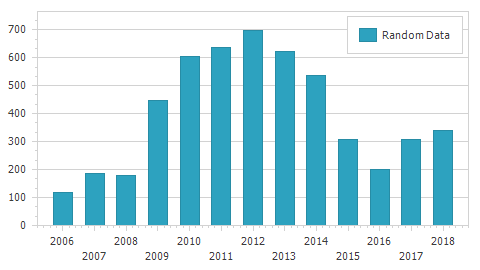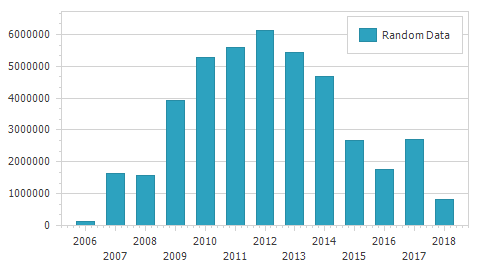Set the CustomAggregateFunction class descendant object to the ScaleGridOptionsBase.CustomAggregateFunction property to use the custom aggregate function. Note that the ScaleGridOptionsBase.AggregateFunction property should be set to Custom.

``````private void Form1_Load(object sender, EventArgs e) {
Series series = chartControl.Series["Random Data"];
series.DataSource = GenerateData(100_000);
series.ArgumentDataMember = "Argument";

XYDiagram diagram = chartControl.Diagram as XYDiagram;
diagram.AxisX.DateTimeScaleOptions.AggregateFunction = AggregateFunction.Custom;
diagram.AxisX.DateTimeScaleOptions.CustomAggregateFunction = new OhlcAggregateFunction();
}

class OhlcAggregateFunction : CustomAggregateFunction {
public override double[] Calculate(GroupInfo groupInfo) {
double open = groupInfo.Values1.First();
double close = groupInfo.Values1.Last();
double high = Double.MinValue;
double low = Double.MaxValue;
foreach (double value in groupInfo.Values1) {
if (high < value) high = value;
if (low > value) low = value;
}

return new double[] { high, low, open, close };
}
}
``````

Handle the ChartControl.AxisScaleChanged event to obtain the axis‘ automatically calculated grid alignment and measurement unit values when the scale mode is automatic. The event arguments also contain the axis itself. This event occurs when the scale mode, measure unit, grid alignment, or grid spacing of the axis scale has been changed.

``````private void Form1_Load(object sender, EventArgs e) {
this.chartControl.AxisScaleChanged += OnAxisScaleChanged;
}

private void OnAxisScaleChanged(object sender, AxisScaleChangedEventArgs e) {
AxisX axis = e.Axis as AxisX;
DateTimeScaleChangedEventArgs args = e as DateTimeScaleChangedEventArgs;
if ((args == null) || (args == null)) return;
axis.Title.Text = String.Format(
"The Axis Grid Alignment Unit is {0}\r\nThe Axis Measure Unit is {1}",
args.GridAlignmentChange.NewValue,
args.MeasureUnitChange.NewValue);
}
``````

When the Aggregate function is set to None, all series are grouped against point arguments and neither aggregate function is applied to data. This means that all points that have the same argument are displayed one over one. Set the ScaleOptionsBase.ScaleMode property to Continuous to disable aggregation.

Important

When a series uses a summary function, the X-axis can’t apply an aggregate function to data.

## Qualitative Data Aggregation Specificity

The Chart Control aggregates qualitative data as follows: if several data rows have the same argument value, they are aggregated. The Chart Control does not aggregate qualitative data by default because the aggregate function is set to None. The following table contains the disabled and enabled aggregation samples:

AxisX.QualitativeScaleOptions.AggregateFunction = AggregateFunction.None; AxisX.QualitativeScaleOptions.AggregateFunction = AggregateFunction.Average;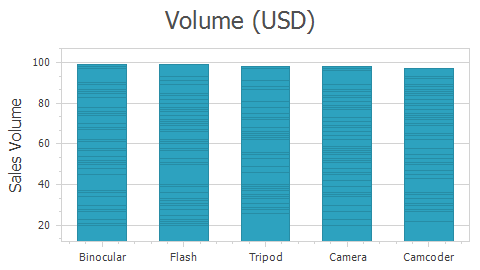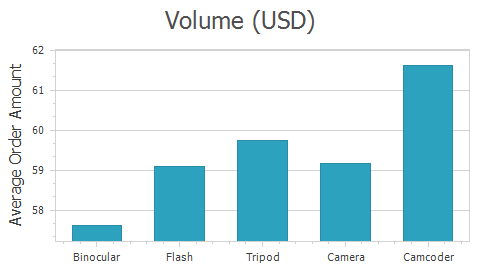## Numeric Data Aggregation Specificity

The Chart Control does not aggregate numeric data by default because the numeric scale mode is set to ScaleMode.Continuous: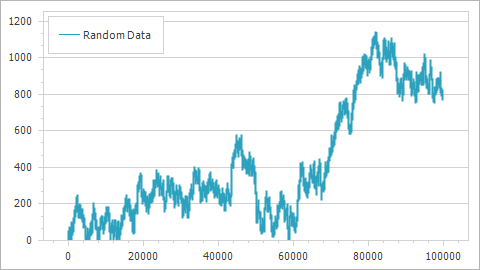The NumericMeasureUnit enumeration lists the values that the NumericScaleOptions.MeasureUnit property uses in the ScaleMode.Manual scale mode. The following table shows the difference between the predefined Hundreds and Thousands measurement units:

NumericScaleOptions.MeasureUnit = NumericMeasureUnit.Hundreds NumericScaleOptions.MeasureUnit = NumericMeasureUnit.Thousands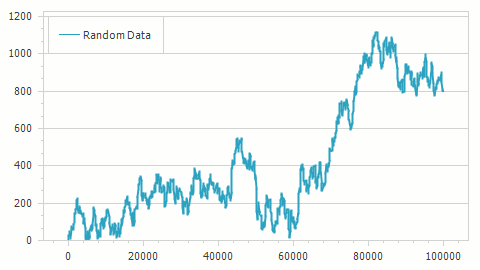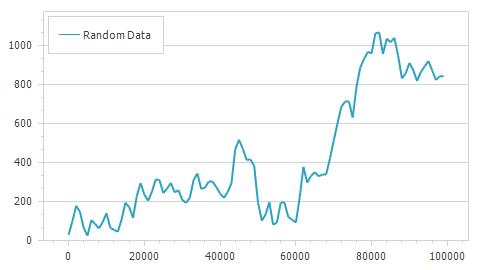If predefined measurement units are not applicable to the specified data source, use the NumericScaleOptions.CustomMeasureUnit property to specify a custom numeric measurement unit.

To use a custom Numeric measure unit for an Automatic scale mode, assign an object of a class implementing the INumericMeasureUnitsCalculator interface to the NumericScaleOptions.AutomaticMeasureUnitsCalculator property of AxisBase.NumericScaleOptions.

``````    private void Form1_Load(object sender, EventArgs e) {

XYDiagram diagram = chart.Diagram as XYDiagram;
if (diagram == null) return;

diagram.AxisX.NumericScaleOptions.AggregateFunction = AggregateFunction.Average;
diagram.AxisX.NumericScaleOptions.ScaleMode = ScaleMode.Automatic;
diagram.AxisX.NumericScaleOptions.AutomaticMeasureUnitsCalculator = new CustomNumericMeasureUnitCalculator();

diagram.AxisY.WholeRange.AlwaysShowZeroLevel = false;
}
class CustomNumericMeasureUnitCalculator : INumericMeasureUnitsCalculator {
public double CalculateMeasureUnit(
IEnumerable<Series> series,
double axisLength,
int pixelsPerUnit,
double visualMin,
double visualMax,
double wholeMin,
double wholeMax) {
double visualRange = visualMax - visualMin;
return Math.Ceiling(visualRange / 20);
}
}
``````

## Date-time Data Aggregation Specificity

The X-axis’s data aggregation is enabled by default because the date-time ScaleOptionsBase.ScaleMode is set to ScaleMode.Manual. In this mode, the DateTimeScaleOptions.GridAlignment and DateTimeScaleOptions.MeasureUnit properties that define the Chart control’s grid appearance are available. The DateTimeScaleOptions.MeasureUnit also configures default axis labels‘ text format: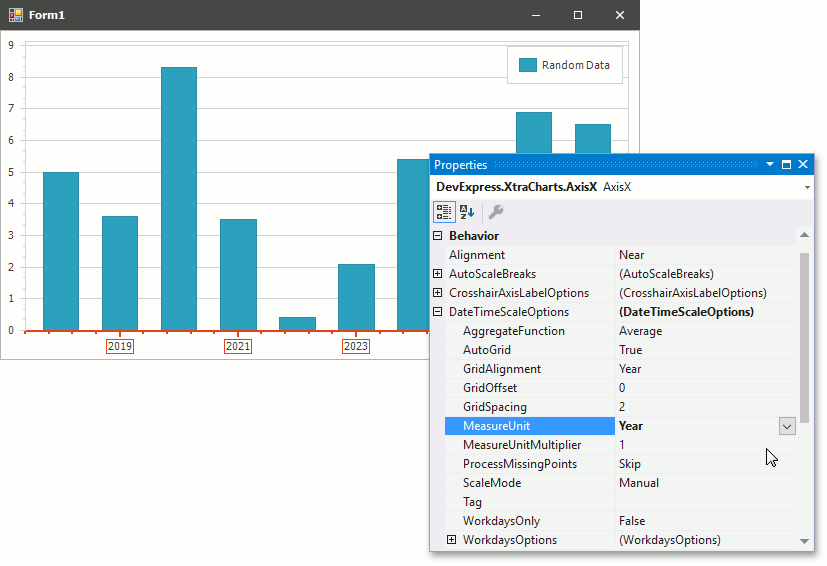The DateTimeScaleOptions.MeasureUnit and DateTimeScaleOptions.MeasureUnitMultiplier properties determines the detail level for date-time values in the Manual scale mode. The DateTimeMeasureUnit enumeration stores predefined measurement units. The following table shows data aggregated by DateTimeMeasureUnit.Quarter and DateTimeMeasureUnit.Year.

DateTimeScaleOptions.MeasureUnit = DateTimeMeasureUnit.Quarter DateTimeScaleOptions.MeasureUnit = DateTimeMeasurementUnit.Year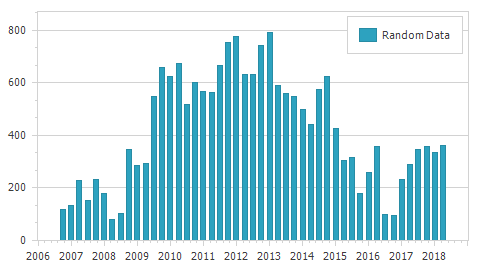To use a custom Date-Time measure unit for an Automatic scale mode, assign an object of a class implementing the IDateTimeMeasureUnitsCalculator interface to the DateTimeScaleOptions.AutomaticMeasureUnitsCalculator property of AxisBase.DateTimeScaleOptions.

``````    private void Form1_Load(object sender, EventArgs e) {

XYDiagram diagram = chart.Diagram as XYDiagram;
if (diagram == null) return;

diagram.AxisX.DateTimeScaleOptions.AggregateFunction = AggregateFunction.Average;
diagram.AxisX.DateTimeScaleOptions.ScaleMode = ScaleMode.Automatic;
diagram.AxisX.DateTimeScaleOptions.AutomaticMeasureUnitsCalculator = new CustomDateTimeMeasureUnitsCalculator();

diagram.AxisY.WholeRange.AlwaysShowZeroLevel = false;
}
class CustomDateTimeMeasureUnitsCalculator : IDateTimeMeasureUnitsCalculator {
const int daysInWeek = 7;
const int daysInMonth = 30;
const int daysInQuarter = 4 * daysInMonth;
const int daysInYear = 365;

const int minCount = 5;

public DateTimeMeasureUnit CalculateMeasureUnit(
IEnumerable<Series> series,
double axisLength,
int pixelsPerUnit,
double visualMin,
double visualMax,
double wholeMin,
double wholeMax) {
// Calculate visual range in msecs.
double visualRange = visualMax - visualMin;
TimeSpan ts = TimeSpan.FromMilliseconds(visualRange);
if (ts.TotalDays >= 1.0d) {
if (ts.TotalDays <= minCount * daysInWeek)
return DateTimeMeasureUnit.Day;
if (ts.TotalDays <= minCount * daysInMonth)
return DateTimeMeasureUnit.Week;
if (ts.TotalDays <= minCount * daysInQuarter)
return DateTimeMeasureUnit.Month;
if (ts.TotalDays <= minCount * daysInYear)
return DateTimeMeasureUnit.Quarter;
else
return DateTimeMeasureUnit.Year;
}
else if (ts.TotalHours >= 20.0d)
return DateTimeMeasureUnit.Hour;
else if (ts.TotalMinutes >= 20.0d)
return DateTimeMeasureUnit.Minute;
else if (ts.TotalSeconds >= 20.0d)
return DateTimeMeasureUnit.Second;
else
return DateTimeMeasureUnit.Millisecond;
}
}
``````